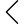182 products found
• s
• m
• l
• xl
• s
• m
• l
• xl
• s
• m
• l
• xl
• s
• m
• l
• xl
• xs
• s
• m
• l
• xl
• s
• m
• l
• xl
• s
• m
• l
• xl
• s
• m
• l
• xl
• s
• m
• l
• xl
• s
• m
• l
• xl
• s
• m
• l
• xl
• s
• m
• l
• xl
• s-m
• l-xl
• s-m
• l-xl
• s
• m
• l
• xl
• s
• m
• l
• xl
• s
• m
• l
• xl
• s
• m
• l
• xl
• s
• m
• l
• xl
• s
• m
• l
• xl
• s
• m
• l
• xl
• s
• m
• l
• xl
• s
• m
• l
• xl
• s
• m
• l
• xl
• s
• m
• l
• xl
• s
• m
• l
• xl
• s
• m
• l
• xl
• s
• m
• l
• xl
• s
• m
• l
• xl
• s
• m
• l
• xl
• s
• m
• l
• xl
• s
• m
• l
• xl
• s
• m
• l
• xl
• s
• m
• l
• xl
• s
• m
• l
• xl
• s
• m
• l
• xl
• s
• m
• l
• xl
• s
• m
• l
• xl
• s
• m
• l
• xl
• s
• m
• l
• xl
•• 1
• 2
• 3
• 5